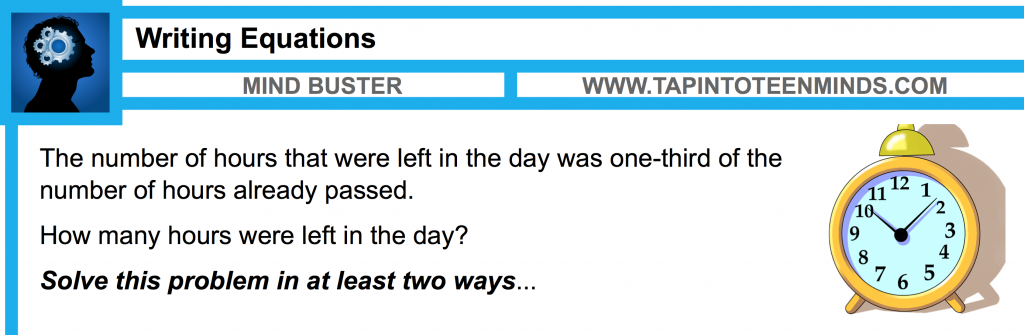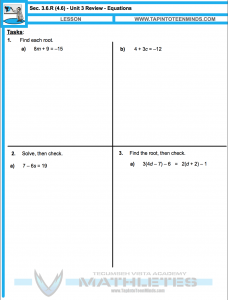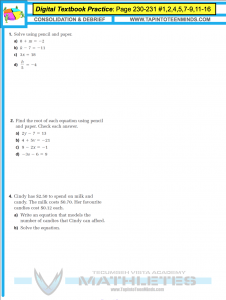# MPM1D – 3.6.R – Unit 3 Solving Equations Review

#### Expectations Covered This Unit:

• simplify numerical expressions involving integers and rational numbers, with and without the use of technology;
• add and subtract polynomials with up to two variables [e.g., (2x – 5) + (3x + 1), (3x2y + 2xy2) + (4x2y – 6xy2)], using a variety of tools (e.g., algebra tiles, computer algebra systems, paper and pencil);
• multiply a polynomial by a monomial involving the same variable [e.g., 2x(x + 4), 2×2(3×2 – 2x + 1)], using a variety of tools (e.g., algebra tiles, diagrams, computer algebra systems, paper and pencil);
• expand and simplify polynomial expressions involving one variable [e.g., 2x(4x + 1) – 3x(x + 2)], using a variety of tools (e.g., algebra tiles, computer algebra systems, paper and pencil);
• solve first-degree equations, including equations with fractional coefficients, using a variety of tools (e.g., computer algebra systems, paper and pencil) and strategies (e.g., the balance analogy, algebraic strategies);
• rearrange formulas involving variables in the first degree, with and without substitution (e.g., in analytic geometry, in measurement);
• solve problems that can be modelled with first-degree equations, and compare algebraic methods to other solution methods.

#### Problems With Homework Form

Students will complete the Problems With Homework Form to indicate any problems they had with the work from the previous day as well as to self-assess where they are in terms of their own learning in the course.

### Minds On

#### Recap and Recall Work From Previous Day

Students will have an opportunity to look at a couple questions from the previous day. Mr. Pearce will record his iPad screen and then post to YouTube after class for student reference.

#### Write and Solve an Equation in at Least Two WaysStudents will work in their table groups to write an equation and solve the equation that could correspond to the following problem:

The number of hours that were left in the day was one-third of the number of hours already passed.

How many hours were left in the day?

Students are instructed to answer the question in at least two ways.

Table groups will share-out via Apple TV to allow students the opportunity to view other ways to solve the problem.

### Action

#### Unit 3 Review – Solving EquationsStudents will continue working collaboratively in their table groups to solve Unit 3 Review – Solving Equations questions.

Each group will be responsible for sharing solutions periodically throughout the duration of the class.

### Consolidate

#### Unit 3 Review ProblemsStudents will consolidate their learning acquired during this lesson as well as throughout the unit by selecting questions from the digital textbook that target problem areas for each student.

Mr. Pearce will display possible consolidation questions over the Apple TV and he will ask students to rank the questions on a scale from 1 to 10, where 1 is easy and 10 is difficult. Students are then suggested to avoid spending too much time on questions that they find “easy” and focus on questions they find moderately to very difficult to ensure they maximize their time spent on math in preparation for the Unit Test next day.

#### MPM1D – 3.6.R – Solving Equations

All Unit 3 Videos can be found on the Math Videos – Unit 3 Solving Equations page.

Here are the two problems we solved at the beginning of class from your last day’s homework…

#### Video #1: Using Equations to Solve Word Problems

Mr. Pearce and students during class solved #10 from the previous day’s homework:

The sum of two consecutive even integers is -134. Find the Numbers.

#### Video #2: Using Equations to Solve Word Problems

The length of Laurie’s rectangular swimming pool is triple its width. The pool covers an area of 192 m^2.

If Laurie swims across the diagonal and back, how far does she travel?